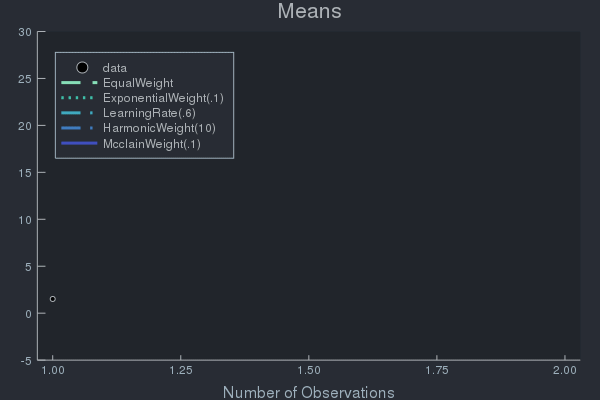# Weights

Many OnlineStats are parameterized by a Weight that controls the influence of new observations. If the OnlineStat is capable of calculating the same result as a corresponding offline estimator, it will have a keyword argument weight. If the OnlineStat uses stochastic approximation, it will have a keyword argument rate (see this great resource on stochastic approximation algorithms).

Consider how weights affect the influence of the next observation on an online mean $\theta^{(t)}$, as many OnlineStats use updates of this form. A larger weight $\gamma_t$ puts higher influence on the new observation $x_t$:

$$$\theta^{(t)} = (1-\gamma_t)\theta^{(t-1)} + \gamma_t x_t$$$
Note

The values produced by a Weight must follow two rules:

1. $\gamma_1 = 1$ (guarantees $\theta^{(1)} = x_1$)
2. $\gamma_t \in (0, 1), \quad \forall t > 1$ (guarantees $\theta^{(t)}$ stays inside a convex space)
Info

The notion of weighting in OnlineStats is fundamentally different than StatsBase.AbstractWeights.

• In OnlineStats, a weight determines the influence of an observation compared to the current state of the statistic.
• In StatsBase, a weight determines the influence of an observation in the overall calculation of the statistic.
julia> using OnlineStats, StatsBaseERROR: ArgumentError: Package StatsBase not found in current path.
- Run import Pkg; Pkg.add("StatsBase") to install the StatsBase package.julia> x = 1:99;julia> w = fill(0.1, 99);julia> # StatsBase: All weights == 0.1
mean(x) ≈ mean(x, aweights(w)) ≈ mean(x, fweights(w)) ≈ mean(x, pweights(w))ERROR: UndefVarError: aweights not definedjulia> # OnlineStats: All weights == 0.1
o = fit!(Mean(weight = n -> 0.1), x)Mean: n=99 | value=90.0003julia> mean(x)  # Every observation has equal influence over statistic.50.0julia> value(o)  # Recent observations have higher influence over statistic.90.00026561398887

## Weight Types

OnlineStatsBase.ExponentialWeightType
ExponentialWeight(λ::Float64)
ExponentialWeight(lookback::Int)

Exponentially weighted observations. Each weight is λ = 2 / (lookback + 1).

ExponentialWeight does not satisfy the usual assumption that γ(1) == 1. Therefore, some statistics have an implicit starting value.

# E.g. Mean has an implicit starting value of 0.
o = Mean(weight=ExponentialWeight(.1))
fit!(o, 10)
value(o) == 1

$γ(t) = λ$

OnlineStatsBase.LearningRateType
LearningRate(r = .6)

Slowly decreasing weight. Satisfies the standard stochastic approximation assumption $∑ γ(t) = ∞, ∑ γ(t)^2 < ∞$ if $r ∈ (.5, 1]$.

$γ(t) = inv(t ^ r)$

## Custom Weighting

The Weight can be any callable object that receives the number of observations as its argument. For example:

• weight = inv will have the same result as weight = EqualWeight().
• weight = x -> .01 will have the same result as weight = ExponentialWeight(.01)
julia> y = randn(100);julia> fit!(Mean(weight = EqualWeight()), y)Mean: n=100 | value=0.000430232julia> fit!(Mean(weight = inv), y)Mean: n=100 | value=0.000430232

## Example of Weight Effects using Data with Concept Drift